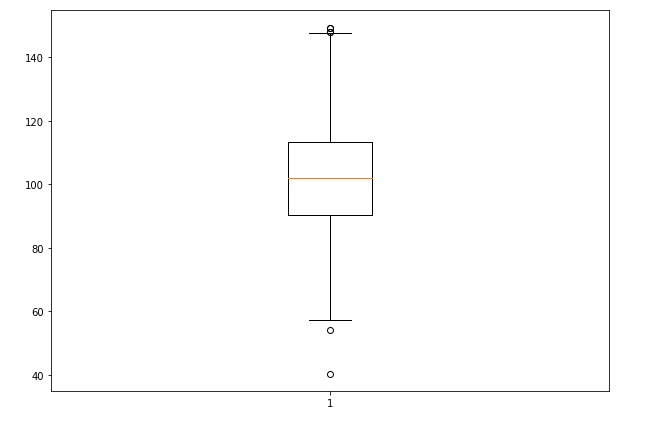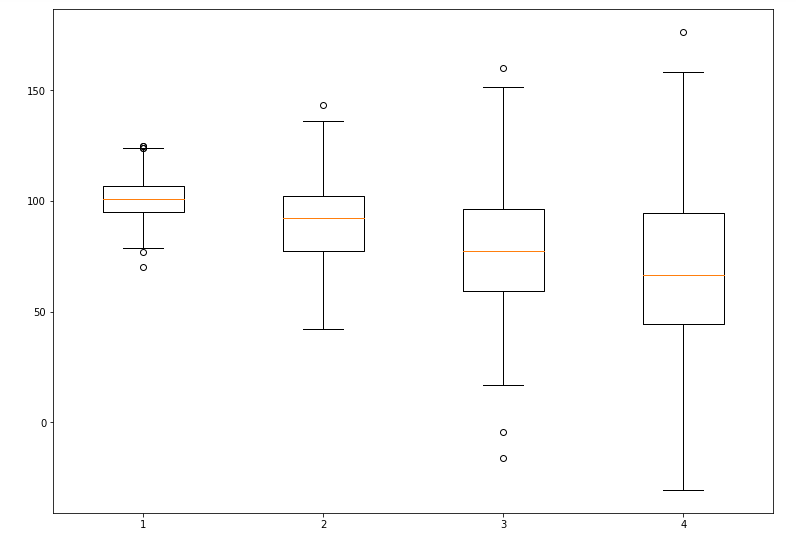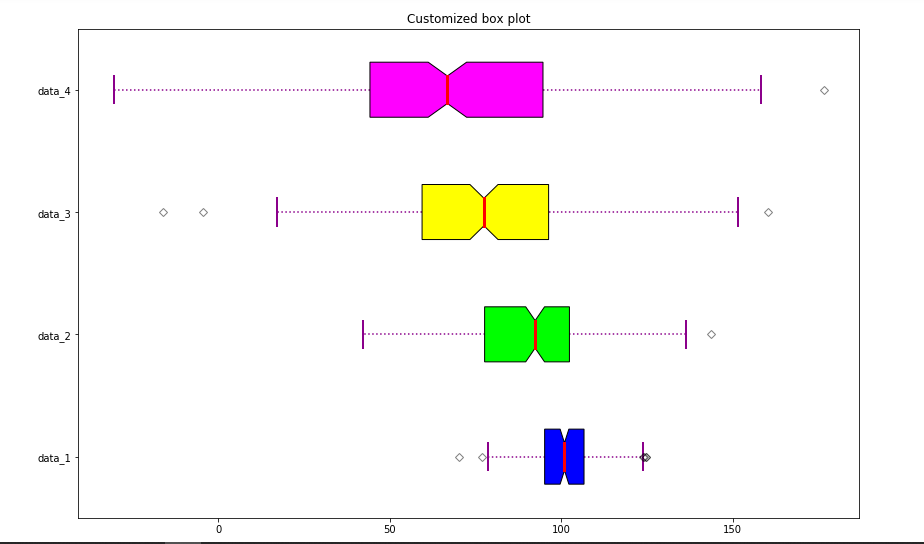# Box Plot in Python using Matplotlib

A Box Plot is also known as Whisker plot is created to display the summary of the set of data values having properties like minimum, first quartile, median, third quartile and maximum. In the box plot, a box is created from the first quartile to the third quartile, a verticle line is also there which goes through the box at the median. Here x-axis denotes the data to be plotted while the y-axis shows the frequency distribution.

## Creating Box Plot

The `matplotlib.pyplot` module of matplotlib library provides `boxplot()` function with the help of which we can create box plots.

Syntax:

matplotlib.pyplot.boxplot(data, notch=None, vert=None, patch_artist=None, widths=None)

Parameters:

Attribute Value
data array or aequence of array to be plotted
notch optional parameter accepts boolean values
vert optional parameter accepts boolean values false and true for horizontal and vertical plot respectively
bootstrap optional parameter accepts int specifies intervals around notched boxplots
usermedians optional parameter accepts array or sequnce of array dimension compatible with data
positions optional parameter accepts array and sets the position of boxes
widths optional parameter accepts array and sets the width of boxes
patch_artist optional parameter having boolean values
labels sequence of strings sets label for each dataset
meanline optinal having boolean value try to render meanline as full width of box
zorder optional parameter sets the zorder of the boxplot

The data values given to the `ax.boxplot()` method can be a Numpy array or Python list or Tuple of arrays. Let us create the box plot by using `numpy.random.normal()` to create some random data, it takes mean, standard deviation, and the desired number of values as arguments.

Example:

 `# Import libraries ` `import` `matplotlib.pyplot as plt ` `import` `numpy as np ` ` `  ` `  `# Creating dataset ` `np.random.seed(``10``) ` `data ``=` `np.random.normal(``100``, ``20``, ``200``) ` ` `  `fig ``=` `plt.figure(figsize ``=``(``10``, ``7``)) ` ` `  `# Creating plot ` `plt.boxplot(data) ` ` `  `# show plot ` `plt.show() `

Output:## Customizing Box Plot

The `matplotlib.pyplot.boxplot()` provides endless customization possibilities to the box plot. The `notch = True` attribute creates the notch format to the box plot, `patch_artist = True` fills the boxplot with colors, we can set different colors to different boxes.The `vert = 0` attribute creates horizontal box plot. `labels` takes same dimensions as the number data sets.

Example 1:

 `# Import libraries ` `import` `matplotlib.pyplot as plt ` `import` `numpy as np ` ` `  ` `  `# Creating dataset ` `np.random.seed(``10``) ` ` `  `data_1 ``=` `np.random.normal(``100``, ``10``, ``200``) ` `data_2 ``=` `np.random.normal(``90``, ``20``, ``200``) ` `data_3 ``=` `np.random.normal(``80``, ``30``, ``200``) ` `data_4 ``=` `np.random.normal(``70``, ``40``, ``200``) ` `data ``=` `[data_1, data_2, data_3, data_4] ` ` `  `fig ``=` `plt.figure(figsize ``=``(``10``, ``7``)) ` ` `  `# Creating axes instance ` `ax ``=` `fig.add_axes([``0``, ``0``, ``1``, ``1``]) ` ` `  `# Creating plot ` `bp ``=` `ax.boxplot(data) ` ` `  `# show plot ` `plt.show() `

Output:Example 2: Let’s try to modify the above plot with some of the customizations:

 `# Import libraries ` `import` `matplotlib.pyplot as plt ` `import` `numpy as np ` ` `  `# Creating dataset ` `np.random.seed(``10``) ` `data_1 ``=` `np.random.normal(``100``, ``10``, ``200``) ` `data_2 ``=` `np.random.normal(``90``, ``20``, ``200``) ` `data_3 ``=` `np.random.normal(``80``, ``30``, ``200``) ` `data_4 ``=` `np.random.normal(``70``, ``40``, ``200``) ` `data ``=` `[data_1, data_2, data_3, data_4] ` ` `  `fig ``=` `plt.figure(figsize ``=``(``10``, ``7``)) ` `ax ``=` `fig.add_subplot(``111``) ` ` `  `# Creating axes instance ` `bp ``=` `ax.boxplot(data, patch_artist ``=` `True``, ` `                ``notch ``=``'True'``, vert ``=` `0``) ` ` `  `colors ``=` `[``'#0000FF'``, ``'#00FF00'``,  ` `          ``'#FFFF00'``, ``'#FF00FF'``] ` ` `  `for` `patch, color ``in` `zip``(bp[``'boxes'``], colors): ` `    ``patch.set_facecolor(color) ` ` `  `# changing color and linewidth of ` `# whiskers ` `for` `whisker ``in` `bp[``'whiskers'``]: ` `    ``whisker.``set``(color ``=``'#8B008B'``, ` `                ``linewidth ``=` `1.5``, ` `                ``linestyle ``=``":"``) ` ` `  `# changing color and linewidth of ` `# caps ` `for` `cap ``in` `bp[``'caps'``]: ` `    ``cap.``set``(color ``=``'#8B008B'``, ` `            ``linewidth ``=` `2``) ` ` `  `# changing color and linewidth of ` `# medians ` `for` `median ``in` `bp[``'medians'``]: ` `    ``median.``set``(color ``=``'red'``, ` `               ``linewidth ``=` `3``) ` ` `  `# changing style of fliers ` `for` `flier ``in` `bp[``'fliers'``]: ` `    ``flier.``set``(marker ``=``'D'``, ` `              ``color ``=``'#e7298a'``, ` `              ``alpha ``=` `0.5``) ` `     `  `# x-axis labels ` `ax.set_yticklabels([``'data_1'``, ``'data_2'``,  ` `                    ``'data_3'``, ``'data_4'``]) ` ` `  `# Adding title  ` `plt.title(``"Customized box plot"``) ` ` `  `# Removing top axes and right axes ` `# ticks ` `ax.get_xaxis().tick_bottom() ` `ax.get_yaxis().tick_left() ` `     `  `# show plot ` `plt.show(bp) `

Output:Attention geek! Strengthen your foundations with the Python Programming Foundation Course and learn the basics.

To begin with, your interview preparations Enhance your Data Structures concepts with the Python DS Course.

My Personal Notes arrow_drop_upCheck out this Author's contributed articles.

If you like GeeksforGeeks and would like to contribute, you can also write an article using contribute.geeksforgeeks.org or mail your article to contribute@geeksforgeeks.org. See your article appearing on the GeeksforGeeks main page and help other Geeks.

Please Improve this article if you find anything incorrect by clicking on the "Improve Article" button below.

Article Tags :

Be the First to upvote.

Please write to us at contribute@geeksforgeeks.org to report any issue with the above content.